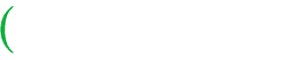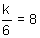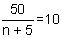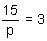# Writing Algebraic EquationsProblem: Jeanne has \$17 in her piggy bank. How much money does she need to buy a game that costs \$68?

Solution: Let x represent the amount of money Jeanne needs. Then the following equation can represent this problem:

17 + x = 68

We can subtract 17 from both sides of the equation to find the value of x.

68 - 17 = x

Answer: x = 51, so Jeanne needs \$51 to buy the game.

In the problem above, x is a variable. The symbols 17 + x = 68 form an algebraic equation. Let's look at some examples of writing algebraic equations.

Example 1: Write each sentence as an algebraic equation.

 Sentence Algebraic Equation A number increased by nine is fifteen. y + 9 = 15 Twice a number is eighteen. 2n = 18 Four less than a number is twenty. x - 4 = 20 A number divided by six is eight.Example 2: Write each sentence as an algebraic equation.

 Sentence Algebraic Equation Twice a number, decreased by twenty-nine, is seven. 2t - 29 = 7 Thirty-two is twice a number increased by eight. 32 = 2a + 8 The quotient of fifty and five more than a number is ten.Twelve is sixteen less than four times a number. 12 = 4x - 16

Example 3: Write each sentence as an algebraic equation.

 Sentence Algebraic Equation Eleni is x years old. In thirteen years she will be twenty-four years old. x + 13 = 24 Each piece of candy costs 25 cents. The price of h pieces of candy is \$2.00. 25h = 200 or .25h = 2.00 Suzanne made a withdrawal of d dollars from her savings account. Her old balance was \$350, and her new balance is \$280. 350 - d = 280 A large pizza pie with 15 slices is shared among p students so that each student's share is 3 slices.Summary: An algebraic equation is an equation that includes one or more variables. In this lesson, we learned how to write a sentence as an algebraic equation.

### Exercises

Directions: Select the algebraic equation that correctly represents the given sentence. Select your answer by clicking on its button. Feedback to your answer is provided in the RESULTS BOX. If you make a mistake, choose a different button.

1.  Twice a number is twenty-eight.

x + 2 = 28
 28 = x 2
2x = 28
None of the above.
 RESULTS BOX:
2.  The quotient of thirty-nine and a number is three.

 39 = 3 w
39w = 3
39 - w = 3
None of the above.
 RESULTS BOX:
3.  Five less than three times a number is forty-six.

5 - 3t = 46
3t - 5 = 46
 5 = 46 3t
None of the above.
 RESULTS BOX:
4.  Three is twenty-one, divided by the sum of a number and five.

3(m+21) = 5
 3 = 21 + 5 m
3m + 5 = 21
None of the above.
 RESULTS BOX:
5.  Eric had \$197 in his savings account before he was paid his weekly salary. His current savings balance is \$429. If Eric deposits all of his earnings, then, how much money does he earn each week?

 197 + 429 = n 197 + n = 429 197n = 429 None of the above.
 RESULTS BOX: